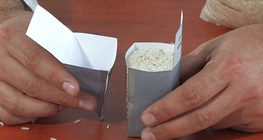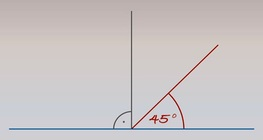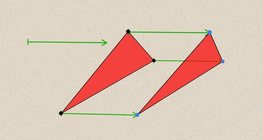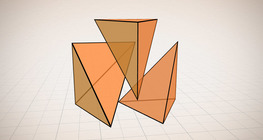Quantity: 0

Total: 0,00

0

# Robots

### Related extras#### The connection between the volume of a square pyramid and the volume of a prism

In this experiment we prove that the volume of the prism is three times the volume of the square...#### Plotting a 45° angle

By bisecting a straight angle (180°), we get a right angle (90°). We get a 45° angle by...#### Geometric transformations – translation

This animation demonstrates geometric translation in both plane and space.#### Platonic solids

This animation demonstrates the five regular three-dimensional (or Platonic) solids, the...#### Volume of a tetrahedron

To calculate the volume of a tetrahedron we start by calculating the volume of a prism.#### Measuring the height of a cathedral

We will measure the height of this large cathedral with a set square and some mathematics.#### Geometric transformations – reflection

This animation demonstrates geometric reflection, a type of geometric transformation both...#### Volume and surface area (exercise)

An exercise about the volume and surface area of solids generated from a ´base cube´.